# Natural number facts for kids

Kids Encyclopedia Facts
 Natural numbers example  (0) 1   2   3   4   5   6   7   8   9   10   11 12 13 14 15 16 17 18 19   20   21 22 23 24 25 26 27 28 29   30   31 32 33 34 35 36 37 38 39   40   41 42 43 44 45 46 47 48 49   50   51 52 53 54 55 56 57 58 59   60   61 62 63 64 65 66 67 68 68   70   71 72 73 74 75 76 77 78 79   80   81 82 83 84 85 86 87 88 89   90   91 92 93 94 95 96 97 98 99   100   200   300   400   500   600   700   800   900   1000   2000   3000   4000   5000   6000   7000   8000   9000   10,000   100,000   1,000,000   1,000,000,000   1,000,000,000,000   Numbers less than 0 (such as −1) are not natural numbers.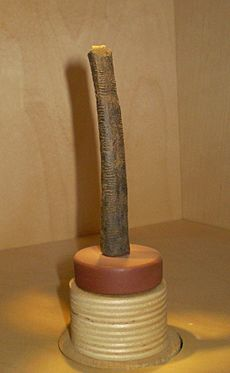The Ishango bone (on exhibition at the Royal Belgian Institute of Natural Sciences) is believed to have been used 20,000 years ago for natural number arithmetic.

Natural numbers, also called counting numbers, are the numbers used for counting things. Sometimes the special number zero is called a natural number. Sometimes one is called the smallest natural number. Natural numbers are always whole numbers (integers) and never less than zero.

There is no largest natural number. The next natural number can be found by adding 1 to the current natural number, producing numbers that go on "for ever". There is no infinite natural number. Any natural number can be reached by adding 1 enough times to the smallest natural number.

## Non-natural numbers

The following types of number are not natural numbers:

• Numbers less than 0 (negative numbers), for example, −2 −1
• Fractions, for example, ½ 3¼
• Decimals, for example, 7.675
• Irrational numbers, for example,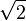${\sqrt{2}}$,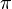$\pi$ (pi)
• Imaginary numbers, for example,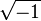${\sqrt{-1}}$ (i)
• infinity, for example,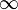$\infty$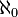$\aleph_0$

## Basic operations

• Addition; The sum of two natural numbers is a natural number.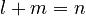$l + m = n$
•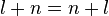$l +n = n + l$
•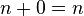$n + 0 = n$
•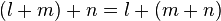$(l + m) + n = l + (m + n)$
• Multiplication": The product of two natural numbers is a natural number.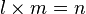$l \times m = n$
•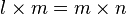$l \times m = m \times n$
•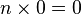$n \times 0 = 0$
•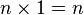$n \times 1 = n$
•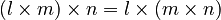$(l \times m) \times n = l \times (m \times n)$
•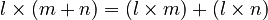$l \times (m + n) = (l \times m) + (l \times n)$
• Ordering: Of two natural numbers, if they are not the same, then one is bigger than the other, and the other is smaller. m = n or m > n or m < n
• if l > m then l + n > m + n and l x n > l x m
• Zero is the smallest natural number: 0 = n or 0 < n
• There is no largest natural number n < n + 1
• "Subtraction": If n is smaller than m then m minus n is a natural number. If n < m then m - n = p.
• if l - m = n then l = n + m
• if n is greater than m, then m minus n is not a natural number
• if l = m - n and p < n then l > m - p
• Division: If$l \times m = n$ then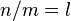$n / m = l$
• Mathematical induction: If these two things are true of any property P of natural numbers, then P is true of every natural number
1. P is true of 0
2. if P of n then P of n+1

## Special natural numbers

• Even numbers: If n = m x 2, then n is an even number
• The even numbers are 0, 2, 4, 6, and so on. Zero is the smallest (or first) even number.
• Odd numbers: If n = m x 2 +1, then n is an odd number
• A number is either even or odd but not both
• The odd numbers are 1, 3, 5, 7, and so on.
• Composite numbers: If n = m x l, and m and l are not 0 or 1, then n is a composite number.
• The composite numbers are 4, 6, 8, 9, 10, 12, 14, 15,16,18,21 and so on.
• Prime numbers: If a number is not 0, 1, and not a composite number, then it is a prime number
• The prime numbers are 2, 3, 5, 7, 11, 13, 17, and so on. Two is the smallest (or first) prime number. Two is the only even prime number.
• There is no biggest prime number.
• Square numbers: If n = m x m, then n is a square. n is the square of m.
• The squares are 0, 1, 4, 9, 16, 25,36,49 and so on.

## How to write it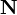$\mathbf{N}$ or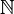$\mathbb{N}$ is the way to write the set of all natural numbers. Because some people say 0 is a natural number, and some people say it is not, people use the following symbols to talk about the natural numbers:

Symbol Meaning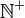$\mathbb{N}^+$ Positive numbers, without zero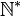$\mathbb{N}^*$ Positive numbers without zero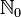$\mathbb{N}_0$ Positive numbers, with zero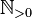$\mathbb{N}_{>0}$ Positive numbers without zero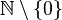$\mathbb{N} \setminus \{0\}$ Positive numbers without zero

## Related pagesNatural number Facts for Kids. Kiddle Encyclopedia.